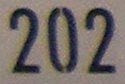## Wednesday, November 10, 2010

### 202

202 = 2 x 101.

202 has a representation as a sum of two squares: 202 = 92 + 112.

202 is the sum of four consecutive primes: 202 = 43 + 47 + 53 + 59.

202 is a semiprime palindrome equal to (2 + 3 + 5 + 7)2 - (22 + 32 + 52 + 72).

202293 begins with the digits 293 (a prime). Note that 293202 begins with the digits 202.202 is the telephone area code for Washington, D.C.